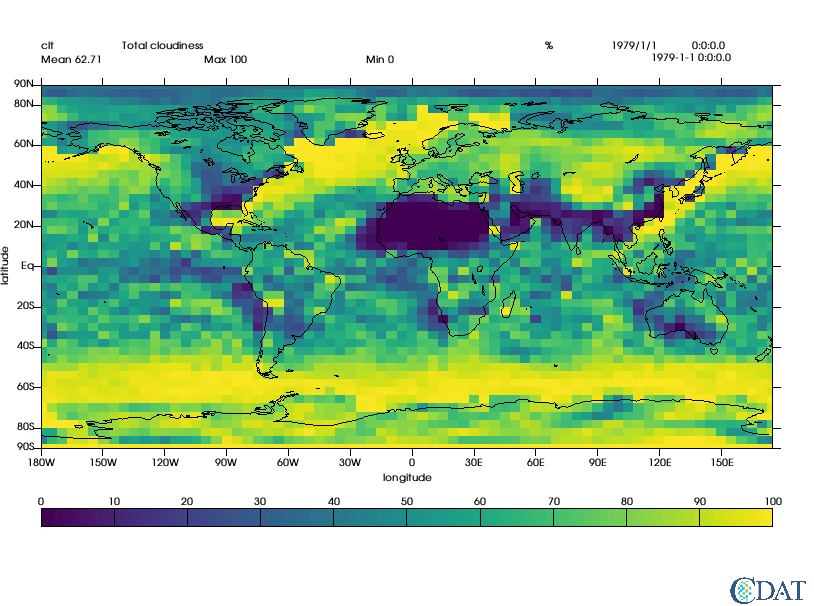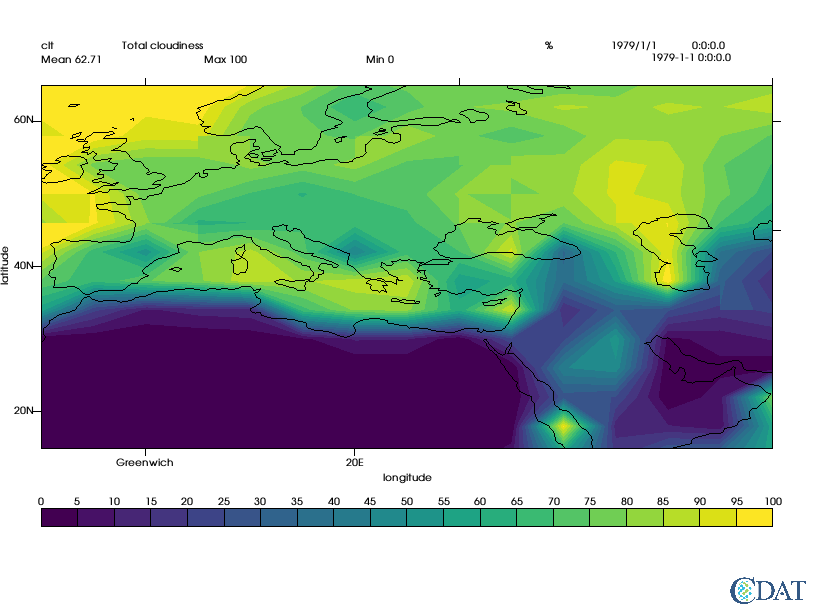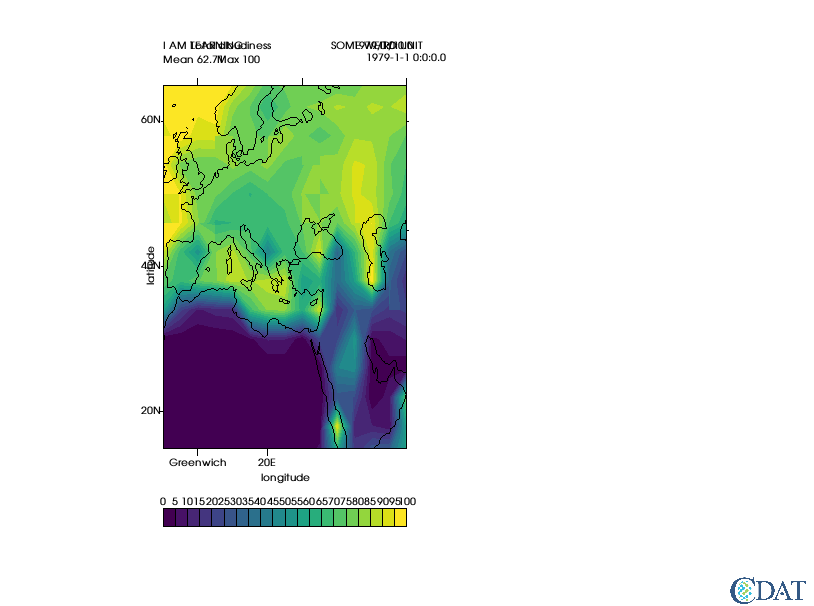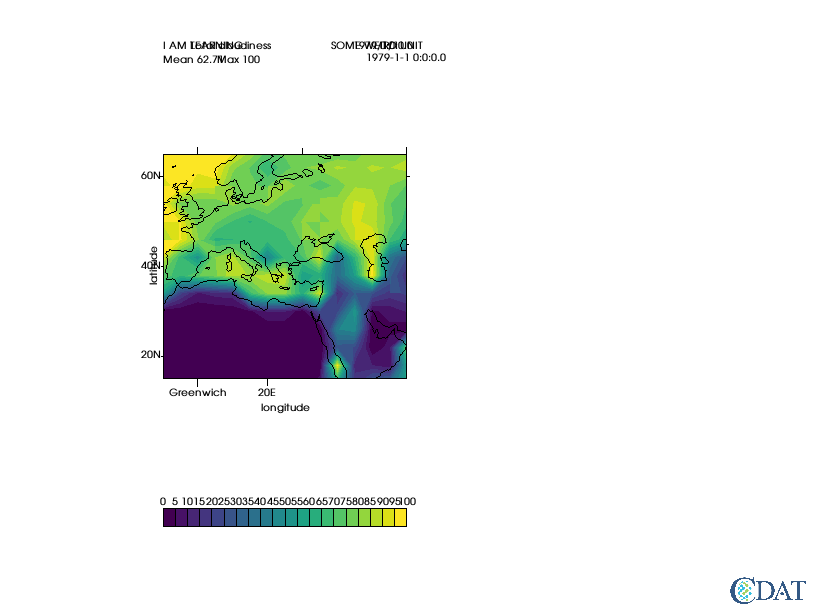# Basic VCS Tutorial¶

In :
import vcs
import cdms2

In :
# MAke sure sample data is here
vcs.download_sample_data_files()

Downloading: 'th_yr.nc' from 'https://uvcdat.llnl.gov/cdat/sample_data/' in: /Users/doutriaux1/anaconda2/envs/cdat/share/uvcdat/sample_data/th_yr.nc
Downloading: 'th_yr.nc' from 'https://uvcdat.llnl.gov/cdat/sample_data/' in: /Users/doutriaux1/anaconda2/envs/cdat/share/uvcdat/sample_data/th_yr.nc
Downloading: 'th_yr.nc' from 'https://uvcdat.llnl.gov/cdat/sample_data/' in: /Users/doutriaux1/anaconda2/envs/cdat/share/uvcdat/sample_data/th_yr.nc

In :
f=cdms2.open(vcs.sample_data+"/clt.nc")

In :
# LOAS SAMPLE DATA
s=f("clt")

In :
# Create vcs canvas (basically X window to draw in)
x=vcs.init(bg=True)

In :
# plot data as is

In :
#because we are in jupyter using bg=True to plot thing in background (no flashing on your screen)
bg=True
x.plot(s)

/Users/doutriaux1/anaconda2/envs/cdat/lib/python2.7/site-packages/vtk/util/numpy_support.py:135: FutureWarning: Conversion of the second argument of issubdtype from complex to np.complexfloating is deprecated. In future, it will be treated as np.complex128 == np.dtype(complex).type.
assert not numpy.issubdtype(z.dtype, complex), \

Out:In :
# Now let's explore some graphics methods available
print vcs.listelements()

['1d', '3d_dual_scalar', '3d_scalar', '3d_vector', 'boxfill', 'colormap', 'display', 'fillarea', 'font', 'fontNumber', 'isofill', 'isoline', 'line', 'list', 'marker', 'meshfill', 'projection', 'scatter', 'streamline', 'taylordiagram', 'template', 'textcombined', 'textorientation', 'texttable', 'vector', 'xvsy', 'xyvsy', 'yxvsx']

In :
# Let's create a isofill
gm = vcs.createisofill()

In :
# Let's see what we can set/do with this
gm.list()

---------- Isofill (Gfi) member (attribute) listings ----------
graphics method = Gfi
name = __isofill_868519049142806
projection = linear
xticlabels1 = *
xticlabels2 = *
xmtics1 =
xmtics2 =
yticlabels1 = *
yticlabels2 = *
ymtics1 =
ymtics2 =
datawc_x1 = 1e+20
datawc_y1 =  1e+20
datawc_x2 =  1e+20
datawc_y2 =  1e+20
datawc_timeunits =  days since 2000
datawc_calendar =  135441
xaxisconvert =  linear
yaxisconvert =  linear
missing =  (0.0, 0.0, 0.0, 100.0)
ext_1 =  False
ext_2 =  False
fillareastyle =  solid
fillareaindices =  
fillareacolors =  
fillareaopacity =  []
fillareapixelspacing =  None
fillareapixelscale =  None
levels =  ([1.0000000200408773e+20, 1.0000000200408773e+20],)
legend =  None


The Graphic Method Controls How Things are Drawn datawc stands for dataworldcoordinates x/yticlabels are the labels to useon x/y axes (python dictionaries {location_value:"string"} x/ymtics are the ticks w/o strings missing is color to use for misssing values (index in colormap, (r,g,b,o) or "string") fillarea are the contour properties

In :
gm.datawc_x1 = -10
gm.datawc_x2 = 60
gm.datawc_y1= 15
gm.datawc_y2 = 65

gm.xticlabels1 = {0:"Greenwich", 20:"20E"}
gm.yticlabels2 = {0:"Equator",60:"Arctic Circle", 45:"45N"}

levels = range(0,101,5) # iso contours to use
colors = vcs.getcolors(levels) # automatically picks colors spread accross your color map
gm.levels = levels
gm.fillareacolors = colors

x.clear()
x.plot(s,gm,bg=bg)

Out:In :
# now to control the LOCATION of elements we use a *template*
t = vcs.createtemplate()

In :
# t.list() would list all properties we can set
t.list()

---------- Template (P) member (attribute) listings ----------
method = P
name = __template_467292516430798
orientation = 0
member =  file
priority = 1
x = 0.0500000007451
y = 0.0130000002682
texttable = default
textorientation = default
member =  function
priority = 1
x = 0.0500000007451
y = 0.0130000002682
texttable = default
textorientation = default
member =  logicalmask
priority = 1
x = 0.0500000007451
y = 0.0329999998212
texttable = default
textorientation = default
member =  transformation
priority = 1
x = 0.0500000007451
y = 0.0529999993742
texttable = default
textorientation = default
member =  source
priority = 1
x = 0.0500000007451
y = 0.941999971867
texttable = default
textorientation = default
member =  dataname
priority = 1
x = 0.0500000007451
y = 0.922999978065
texttable = default
textorientation = default
member =  title
priority = 1
x = 0.15000000596
y = 0.922999978065
texttable = default
textorientation = default
member =  units
priority = 1
x = 0.670000016689
y = 0.922999978065
texttable = default
textorientation = default
member =  crdate
priority = 1
x = 0.75
y = 0.922999978065
texttable = default
textorientation = default
member =  crtime
priority = 1
x = 0.850000023842
y = 0.922999978065
texttable = default
textorientation = default
member =  comment1
priority = 1
x = 0.10000000149
y = 0.954999983311
texttable = default
textorientation = default
member =  comment2
priority = 1
x = 0.10000000149
y = 0.975000023842
texttable = default
textorientation = default
member =  comment3
priority = 1
x = 0.10000000149
y = 0.995000004768
texttable = default
textorientation = default
member =  comment4
priority = 1
x = 0.10000000149
y = 0.999000012875
texttable = default
textorientation = default
member =  xname
priority = 1
x = 0.5
y = 0.21
texttable = default
textorientation = defcenter
member =  yname
priority = 1
x = 0.006
y = 0.56
texttable = default
textorientation = defup
member =  zname
priority = 0
x = 0.0
y = 0.995000004768
texttable = default
textorientation = default
member =  tname
priority = 1
x = 0.0
y = 0.995000004768
texttable = default
textorientation = default
member =  xunits
priority = 1
x = 0.600000023842
y = 0.277000010014
texttable = default
textorientation = default
member =  yunits
priority = 1
x = 0.019999999553
y = 0.658999979496
texttable = default
textorientation = default
member =  zunits
priority = 0
x = 0.0
y = 0.995000004768
texttable = default
textorientation = default
member =  tunits
priority = 0
x = 0.0
y = 0.995000004768
texttable = default
textorientation = default
member =  xvalue
priority = 1
x = 0.800000011921
y = 0.941999971867
format = default
texttable = default
textorientation = default
member =  yvalue
priority = 1
x = 0.800000011921
y = 0.922999978065
format = default
texttable = default
textorientation = default
member =  zvalue
priority = 1
x = 0.800000011921
y = 0.902999997139
format = default
texttable = default
textorientation = default
member =  tvalue
priority = 1
x = 0.800000011921
y = 0.883000016212
format = default
texttable = default
textorientation = default
member =  mean
priority = 1
x = 0.0500000007451
y = 0.899999976158
format = default
texttable = default
textorientation = default
member =  min
priority = 1
x = 0.449999988079
y = 0.899999976158
format = default
texttable = default
textorientation = default
member =  max
priority = 1
x = 0.25
y = 0.899999976158
format = default
texttable = default
textorientation = default
member =  xtic1
priority = 1
y1 = 0.259999990463
y2 = 0.24699999392
line = default
member =  xtic2
priority = 1
y1 = 0.860000014305
y2 = 0.871999979019
line = default
member =  xmintic1
priority = 0
y1 = 0.259999990463
y2 = 0.248999999285
line = default
member =  xmintic2
priority = 0
y1 = 0.860000014305
y2 = 0.868000014305
line = default
member =  ytic1
priority = 1
x1 = 0.0500000007451
x2 = 0.0399999991059
line = default
member =  ytic2
priority = 1
x1 = 0.949999988079
x2 = 0.959999978542
line = default
member =  ymintic1
priority = 0
x1 = 0.0500000007451
x2 = 0.0450000017881
line = default
member =  ymintic2
priority = 0
x1 = 0.949999988079
x2 = 0.954999983311
line = default
member =  xlabel1
priority = 1
y = 0.234999999404
texttable = default
textorientation = defcenter
member =  xlabel2
priority = 0
y = 0.880000004768
texttable = default
textorientation = defcenter
member =  ylabel1
priority = 1
x = 0.0399999991059
texttable = default
textorientation = defright
member =  ylabel2
priority = 0
x = 0.959999978542
texttable = default
textorientation = default
member =  box1
priority = 1
x1 = 0.0500000007451
y1 = 0.259999990463
x2 = 0.949999988079
y2 = 0.860000014305
line = default
member =  box2
priority = 0
x1 = 0.0
y1 = 0.300000011921
x2 = 0.920000016689
y2 = 0.879999995232
line = default
member =  box3
priority = 0
x1 = 0.0
y1 = 0.319999992847
x2 = 0.910000026226
y2 = 0.860000014305
line = default
member =  box4
priority = 0
x1 = 0.0
y1 = 0.0
x2 = 0.0
y2 = 0.0
line = default
member =  line1
priority = 0
x1 = 0.0500000007451
y1 = 0.560000002384
x2 = 0.949999988079
y2 = 0.560000002384
line = default
member =  line2
priority = 0
x1 = 0.5
y1 = 0.259999990463
x2 = 0.5
y2 = 0.860000014305
line = default
member =  line3
priority = 0
x1 = 0.0
y1 = 0.52999997139
x2 = 0.899999976158
y2 = 0.52999997139
line = default
member =  line4
priority = 0
x1 = 0.0
y1 = 0.990000009537
x2 = 0.899999976158
y2 = 0.990000009537
line = default
member =  legend
priority = 1
x1 = 0.0500000007451
y1 = 0.129999995232
x2 = 0.949999988079
y2 = 0.159999996424
line = default
texttable = default
textorientation = defcenter
offset = 0.01
member =  data
priority = 1
x1 = 0.0500000007451
y1 = 0.259999990463
x2 = 0.949999988079
y2 = 0.860000014305


x/y coordinate are in % of page priority is the layer higher means on top of other objects, 0 means turn off

important eleements are dataname, title, mean, max, min, units values come from data plotted

data is the area where to plot the data legend is the area used by the legend/colorbar

see bellow for more info on text objects, but they are basically contrlled via textorientation and texttable objects properties

In :
t.min.priority =0 # turn off min
s.id = "I AM LEARNING" # change dataname
s.title = "THSI IS MY TITLE"
s.units= "SOME WEIRD UNIT"

t.reset('x',.2,.5,t.data.x1,t.data.x2) # reset template to go from 20% to %0% of page (left essentially)

x.clear()
x.plot(s,gm,t)

Out:In :
#Let's preserve the aspect ratio
x.clear()
x.plot(s,gm,t,ratio="autot")

Out: